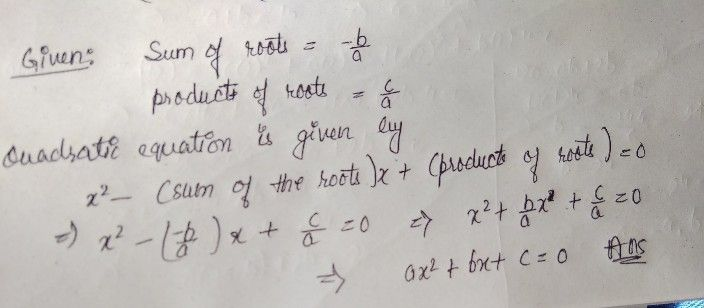Symbol
Problem$111$ 1.The sum of the roots of the quadratic equation $ax^{2}+bx+c=0is\dfrac {-b} {a}$ $2.$ The product of the roots of the quadratic equation $ax^{2}+bx+c=0is\dfrac {c} {a}$ To determine the quadratic equation given the sum and the product of the roots $use3$ $2-$ (the sum of the roots) $\right)$ $x+$ $1$ (the product of the roots) $s\right)=0$
1st-6th grade
Other
Search count: 119
SolutionQanda teacher - khubchandr